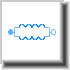Linear - MapleSim Help

Linear

Linear tire model used to determine the forces and moments developed at the tire contact patchThe Linear tire model provides a linear relationship between the slip angle and longitudinal slip of the tire, and the lateral and longitudinal forces developed at the contact patch. The aligning moment, rolling resistance and overturning moment are assumed to be zero.

Note: The $\mathrm{hubFrame}$ port of the Linear tire model component  must be connected directly to the $\mathrm{hubFrame}$ port of a tire body component, as shown in the diagram below.Connections

 Name Description Color $\mathrm{hubFrame}$ Tire reference frame, which must be connected to a tire body component. Gray

Parameters

 Symbol Default Units Description ID ${C}_{\mathrm{long}}$ 115000 $N$ Longitudinal force coefficient Clong ${C}_{\mathrm{lat}}$ 117000 $N$ Lateral force coefficient Clat